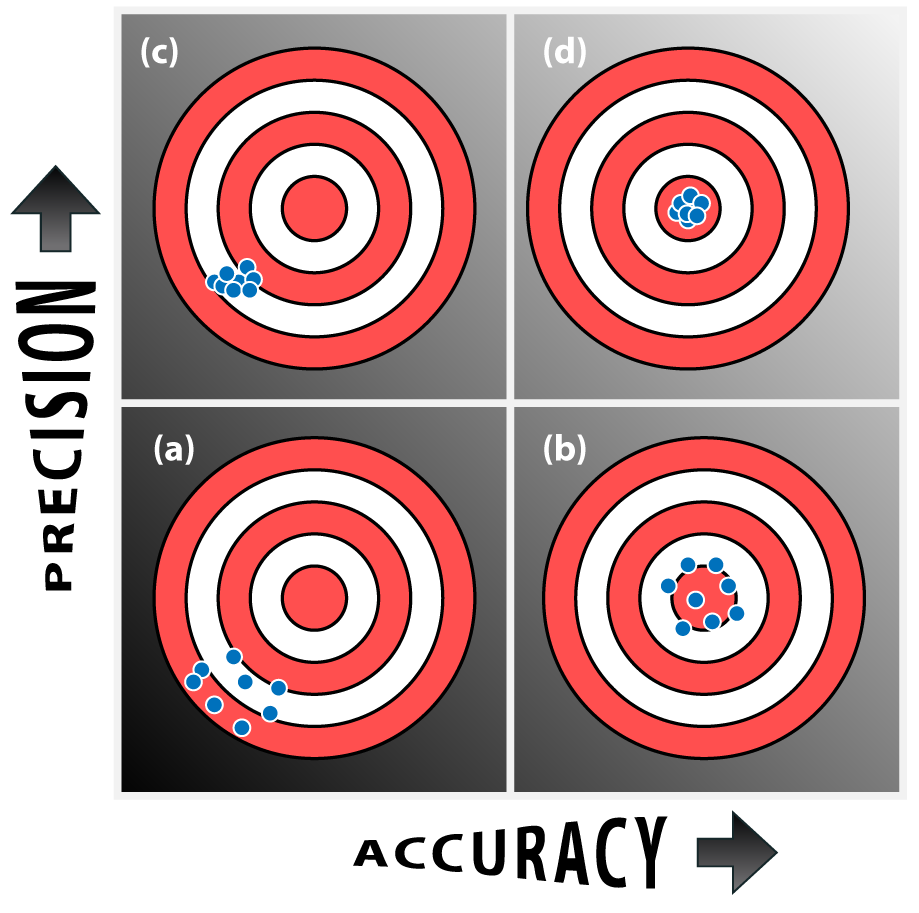# Measurements and Units Accuracy, Precision, and Errors: Accuracy, Precision, and Errors

## Accuracy and Precision

Accuracy and precision are terms often used to describe the reliability of measurements. However, they must be clearly differentiated. Accuracy refers to how close a measured value is to the real or “true” value. Precision refers to the degree of reproducibility of a measured quantity (the closeness of agreement when the same quantity is measured several times–how close the measurements are to each other). This difference is demonstrated in the following illustration:Systematic error (determinate error): Built-in, inherent error. It always occurs in the same direction each time; it is always high or always low. A systematic error can be corrected by proper calibration or running controls or blanks (e.g. a thermometer consistently gives readings that are 2 °C too low). Large systematic errors lower the accuracy of a measurement.

Random error (indeterminate error): A measurement has an equal probability of being too high or too low. It is due to limitations in an experimenter’s skills or ability to read scientific measurements. It cannot be corrected. Large random errors lower the precision of the measurements (e.g., the temperature in room varies “wildly”).

#### EXAMPLE 1: Weigh a piece of brass five times on the analytical balance and obtain the following results:

 Weighing Result 1 2.486 g 2 2.487 g 3 2.485 g 4 2.484 g 5 2.488 g

Average = (2.486 g + 2.487 g + 2.485 g + 2.484 g + 2.488 g) / 5 = 2.486 g

Normally, we would assume that the true mass of the piece of brass is very close to 2.486 g, the average of the five results.

However, if the analytical balance has a defect causing it to be consistently 1.000 g too high (a systematic error of +1.000 g), then the measured value of 2.486 g would be seriously in error.

The point: high precision among several measurements is an indication of accuracy only if systematic errors are absent.

EXAMPLE 2: To check the accuracy of a graduated cylinder, fill the cylinder to the 25 mL mark using water delivered from a burette and then read the volume delivered.

 Trial Volume Shown by Graduated Cylinder  (mL) Volume Shown by Burette (mL) 1 25 26.54 2 25 26.51 3 25 26.60 4 25 26.49 5 25 26.57 Average 25 26.54

The results show good precision (for a graduated cylinder), so  the student has good technique.

However, the average value measured using the burette is significantly different from 25 mL.

Thus, this graduated cylinder is not very accurate. It produces a systematic error (in this case, the result is low for each measurement).

## Ways of Comparing Experimental Values

Used to compare an unknown value with a theoretical value. It is a measure of the degree of accuracy.Used to compare two experimental values which are expected to be the same. It is a measure of the degree of precision. It is also used to compare two values which are not necessarily expected to be the same. (Particularly useful to determine which are the best runs to use for calculations in titration experiments).EXAMPLE: It is necessary to calculate the percentage difference between the best two runs (e.g the ones with the smallest % difference).  In the example below, the best runs to use would be 2 & 3 since their % difference is smallest ( < 1%).

 Runs % Difference 1 & 2 1.79 % 1 & 3 0.97 % 2 & 3 0.82 %

Used to compare two values which are different due to an imposed stress on a system. It is a measure of the degree of the effect caused by the stress.